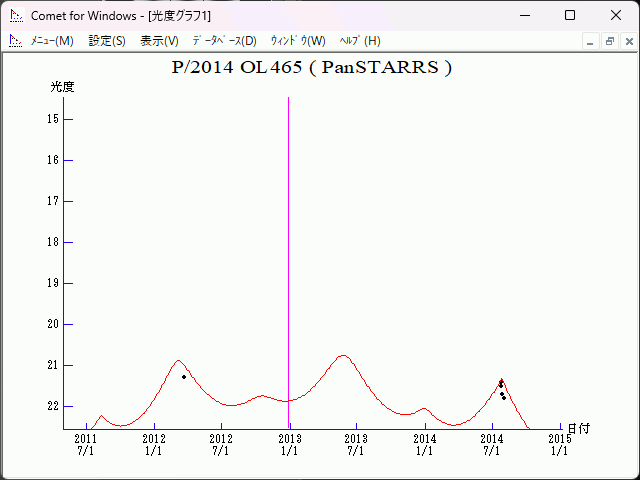# \$B%Q%s%9%?!<%:WB@1(B

464P/PanSTARRS (2012)###\$B%W%m%U%#!<%k(B

 \$BId9f(B 464P/2014 OL465 \$BH/8+F|(B 2014\$BG/(B7\$B7n(B25\$BF|(B \$BH/8+8wEY(B 21.5\$BEy(B \$BH/8+ Pan-STARRS 1 telescope (Haleakala)

###\$B50F;MWAG(B

```                    Epoch = 2012 Dec. 19.0 TT
T = 2012 Dec. 29.13025 TT        Peri. = 267.52913
e = 0.2811155                    Node  = 309.73434 2000.0
q = 3.3685450 AU                 Incl. =  21.66903
a =  4.6857947 AU   n = 0.09716933   P =  10.14 years
```

###\$B8wEYJQ2=(B

```        H = 15.5  G = 0.15
```##### \$B50F;MWAG\$O!"(BCBET 5262\$B\$K7G:\\$5\$l\$?\$b\$N\$G\$9!#(B \$B8wEY%0%i%U\$O(BComet for Windows\$B\$G:n@.\$7\$?\$b\$N\$G\$9!#(B